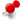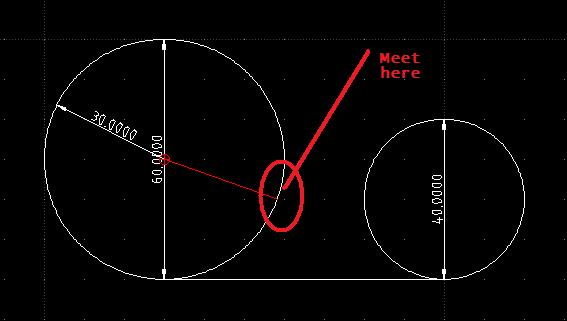# How to draw 2 circle that will meet in their radiusClassicListThreaded8 messagesOpen this post in threaded view
|

## How to draw 2 circle that will meet in their radiusSay I would like to draw two circles with different diameter or radius. As in the picture, say that the diameter (D1) = 60, Diameter 2 (D2) = 40 (hence R1=30, R2=20). So, the distance between their center will be 30+20=50, as they meet exactly in one point. The two circles are drawn above the same plane, say, above a horizontal plane. I tried 4 tangential options from circle drawing menu, but didn't work. Of course I can do it by do some maths. But what I am asking here is how to do without doing that math, just as simple as using that tangential. Best regard,
Open this post in threaded view
|

## Re: How to draw 2 circle that will meet in their radius

 El dj. 09 de 04 de 2020 a les 07:26 -0700, en/na sunggus [via LibreCAD] va escriure: > > > Say I would like to draw two circles with different diameter or > radius. As in the picture, say that the diameter (D1) = 60, Diameter > 2 (D2) = 40 (hence R1=30, R2=20). So, the distance between their > center will be 30+20=50, as they meet exactly in one point. The two > circles are drawn above the same plane, say, above a horizontal > plane. I tried 4 tangential options from circle drawing menu, but > didn't work. Of course I can do it by do some maths. But what I am > asking here is how to do without doing that math, just as simple as > using that tangential. > > Best regard, > What version of LibreCAD?, in what OS? For me it works as following (LibreCAD 2.1.3 on Linux Debian Sid): draw a horizontal line (the plane) draw a tangential cicle to that line (the first circle, here I miss a tangential radius command) click on tangential 2 circles radius, select the circle and the line as first and second circles and write the radius size in the box. Yes, I know it is not obvious to select the line when the command tells you it needs a circle :) HTH Pere
Open this post in threaded view
|

## Re: How to draw 2 circle that will meet in their radius

 draw a tangential circle to that line perepujal wrote El dj. 09 de 04 de 2020 a les 07:26 -0700, en/na sunggus [via LibreCAD] va escriure: > > > Say I would like to draw two circles with different diameter or > radius. As in the picture, say that the diameter (D1) = 60, Diameter > 2 (D2) = 40 (hence R1=30, R2=20). So, the distance between their > center will be 30+20=50, as they meet exactly in one point. The two > circles are drawn above the same plane, say, above a horizontal > plane. I tried 4 tangential options from circle drawing menu, but > didn't work. Of course I can do it by do some maths. But what I am > asking here is how to do without doing that math, just as simple as > using that tangential. > > Best regard, > What version of LibreCAD?, in what OS? For me it works as following (LibreCAD 2.1.3 on Linux Debian Sid): draw a horizontal line (the plane) draw a tangential cicle to that line (the first circle, here I miss a tangential radius command) click on tangential 2 circles radius, select the circle and the line as first and second circles and write the radius size in the box. Yes, I know it is not obvious to select the line when the command tells you it needs a circle :) HTH Pere I use LC 2.1.3 under Windows. But I guess that should not be problem. "Draw a tangential cicle to that line", of course I can do it, but I can not select my own radius. Best regard,
Open this post in threaded view
|

## Re: How to draw 2 circle that will meet in their radius

 For tangential circle to line with radius you can use Circle 2Points (diameter instead of radius, second point @0,60).
Open this post in threaded view
|

## Re: How to draw 2 circle that will meet in their radius

 It is asked to specify the first point of the tangential: "Specify the first point of the tangential circle." That's the thing I don't know (without doing math). Best regard,
Open this post in threaded view
|

## Re: How to draw 2 circle that will meet in their radius

 In reply to this post by dellus What I expect is, by drawing the first circle and the horizontal plane, to do the second circle I just need to put its radius, the CAD will automatically adjust to have 2 tangential points. Best regard,